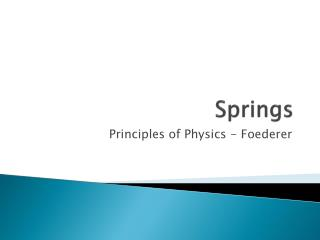DownloadDownload PresentationSprings

# Springs

Télécharger la présentation## Springs

- - - - - - - - - - - - - - - - - - - - - - - - - - - E N D - - - - - - - - - - - - - - - - - - - - - - - - - - -
##### Presentation Transcript

1. Springs Principles of Physics - Foederer

2. Elastic Potential Energy Energy is stored in a spring when work is done to compress or elongate it Compression or elongation = change in length = x (m)

3. Springs have a spring constant (k) that tells how much force is required to change the length of a spring by 1m. Robert Hooke put it all together→ Fs = kx Fs = spring force (N) k = spring constant (N/m) x = change in length (m)

4. Based on Hooke’s experiments, it was determined that the energy stored in the spring can be calculated using the following formula: PEs = ½ kx2 PEs = elastic potential energy (joules) k = spring constant (N/m) x = change in length (m)

5. Example A spring (k = 340 N/m) is compressed 0.02m. How much energy is stored in the spring? PEs = ½ kx2 = ½(340 N/m)(0.02m)2 = 0.068 J

6. Example A 5 kg block sliding on a frictionless horizontal surface with a speed of 5 m/s collides with a spring (k = 120 N/m) and comes to rest. How much will the spring be compressed? KE→PEs ½ mv2 = ½ kx2 ½ (5kg)(5 m/s)2 = ½(120 N/m)(x)2 x = 1.02 m

7. Example The diagram at right shows a 0.1-kilogram apple attached to a branch of a tree 2 meters above a spring on the ground below. The apple falls and hits the spring, compressing it 0.1 meter from its rest position. Assume all energy is conserved within the system. • Write the energy transformations that occur as the apple falls from the tree. ______→ ______→ ______ PE KE PEs

8. Example The apple (0.1 kg) falls 2m and hits the spring, compressing it 0.1 meter from its rest position. Assume all energy is conserved within the system. • Determine the gravitational PE of the apple before it falls. PE= mgh PE = 0.1(9.8)(2) PE = 1.96 J

9. Example The apple (0.1 kg) falls and hits the spring, compressing it 0.1 meter from its rest position. Assume all energy is conserved within the system. • Determine the kinetic energy and the velocity of the apple when it hits the spring. KE = ½ mv2 1.96 = ½ (0.1)(v2) ½ (0.1) ½ (0.1) 39.2 = v2 v = 6.26 m/s √ √

10. Example The apple (0.1 kg) falls and hits the spring, compressing it 0.1 meter from its rest position. Assume all energy is conserved within the system. • Determine the elastic potential energy and the spring constant of this spring? PEs = ½ kx2 1.96 = ½(k)(0.1)2 ½ (0.1)2 ½ (0.1)2 k = 392 N/m